# Science Class 9 Chapter – 8 : Motion | Notes | Study material and PDF download

2
9045Science for Class – IX (NCERT)

Chapter 8 – Motion

8.1- Introduction

Motion, as the word itself says, is the movement of different living and non-living things. We see birds flying, people moving, cars running – all of which are different types of motions that we come across in our everyday life. What can we study about motion? Why is the study of motion included in the study of physics? Let us go through this chapter to understand this.

8.2 – How do we describe motion?

How do we know that something is in motion or not? Before we discuss this topic furthermore, let us talk about two familiar terms that are necessary to know about in this chapter

Motion: The state in which an object (or person) is moving is called the state of motion.

Rest: The state in which an object (or person) is not moving is called the state of rest. So, for an object that is simply lying on the ground, we say that the object is in a state of rest.

Now coming back to the topic, how can we know that an object is moving? Or how can we say that and object is in the state of rest? If we find that the distance of an object from a fixed point is continuously increasing or decreasing, then we might say that the object is moving or the object is in the state of motion. The fixed point about which we are talking is also called the reference point or origin.

Origin: The origin is a reference point which is used to determine the state and location of an object. An object can be near, far, beside, below or above that reference point (or origin).

8.2.1- Motion along a straight line

To understand above points more clearly, we must learn about motion along a straight line. It is the simplest type of motion. To understand this concept more clearly, take the example of an object moving along a straight path.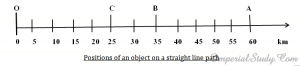Suppose, the object starts its journey from O, which is treated as its reference point or origin. Then A, B and C represent the position of that object at different intervals. Now, first the object moves towards A, passing the points C and B in its way. Then, it moves back along the same path and reaches at C passing through B.

Let us calculate the length that it has covered. It is OA + AC, i.e. 60 km + 35 km = 95 km. Here, in this case, we need to specify only the numerical value of the distance covered by the object in order to calculate it; we don’t need to specify the direction of motion. Many quantities are described by only their numerical value.

The numerical value of a physical quantity is also called its magnitude. Also, there are some terms that may appear many times in this and the next chapter:

Distance: The magnitude of length covered by an object is known as distance.

Initial position: Position of an object when it is at the origin or the position of an object before it has started to move is called its initial position. The word ‘initial’ means starting. So, initial position is the starting position of an object.

Final position: The position of an object after it has completed its movement/completed moving is called its final position. It is the position at which the object has stopped.

Displacement: It is the shortest distance between the initial position and the final position of an object. In this case, the displacement of the object is the distance between O and C i.e. 25 km.

Can the distance and displacement be equal?

The topic that we are going to discuss now is not about finding any relation between the distance and the displacement, but it focuses on the difference between the distance and the displacement.

Suppose, the object moves again from O to B. This time the distance is 35 km and the displacement is also 35 km. In such a simple case, the distance and displacement can be equal. Now, suppose the object moves from B to C. The distance in this case is 35 + 10 km (since B and C are at a distance of 10 km from each other)=45 km while the displacement would be 25 km which is the shortest distance between O and C. Also, suppose that the object returns back to O. The distance now would be 45 + 25 = 70 km. What is the displacement now?

We see that the initial position and the final position of the object are the same i.e. O. So, the displacement now would be zero. So, again the distance and displacement are not equal in this case. Thus we conclude that distance and displacement are two different quantities that are used to determine the overall motion of an object. We also conclude that the displacement can be zero in case the initial position and final position of an object coincide with each other (are at the same point).

8.2.2 – Uniform and Non-uniform motion

In this section, we discuss two categories of motion which are related to its speed, i.e. distance covered in unit time. Let us look at these terms:

Uniform motion: When an object covers equal amounts of distance in equal intervals of time, then it is called the uniform motion. For instance, suppose a man who is walking is able to walk 100 metres in one minute, another 100 metres in second minute and another 100 metres in the third minute, then it is the uniform motion.

Non-uniform motion: When an object covers unequal amounts of distance in equal intervals of time, it is called non-uniform type of motion. For instance, suppose when a car covers a distance of 30 km in one hour, 35 km in second hour and 32 km in third hour, then it is called the non-uniform motion.

8.3- Speed/Rate of motion

We know that different objects may take different amount of time in covering different amount of distance. Some may be slow and some may be fast. This rate at which an object covers the given distance is called its speed.

Also, we must introduce a familiar term, called the SI unit. Many of you may know about this term, but for those who don’t know, SI unit is a unit in which a given quantity can be expressed. To understand this more clearly, look at the table of SI units of familiar quantities that is given below: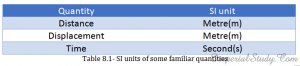We see that distance is usually expressed in metres, so its SI unit is ‘Metre’ or ‘m’ in short. Again, the displacement is nothing but another type of distance, so its SI unit is again ‘Metre’ or ‘m’. In the case of time, its basic unit is second so its SI unit is also ‘Second’ or ‘s’ in short.

Now, speed is also a quantity. So, there is an SI unit of speed too. The SI unit of speed is metre per second or m/s. This is also referred as m s-1. There are some other units of speed like centimetre per second (cm s-1), and kilometre per hour (km h-1). These are not the SI units but these units are commonly used while specifying the speed of an object.

Average speed: The total amount of distance travelled by an object in the total amount of time is known as the average speed of that object. We use average speed because speed of most objects is non-uniform and keeps changing.

Average speed =

Total distance travelled / Total time taken

So, if an object travels a distance s in time t, the speed v is,

v=s/t

For example, suppose a car travels a distance of 120 km in 3 hrs, then its average speed v is 120/3 = 40 km/h. The car might not have travelled at 40 km/h at all the time and sometimes it may have been faster or slower, but this is the average speed of the car.

8.3.1- Speed with direction

When we specify the direction of motion along with its speed, we are referring to its velocity. Velocity is the speed of an object moving in a specific/definite direction. The velocity of an object can be either uniform or variable. The velocity can be changed by changing object’s speed, changing object’s direction of motion or changing both speed and direction of motion.

Average velocity:

If an object is moving along a straight path, we can express the magnitude of its rate of motion in terms of its average velocity.

Suppose the velocity of an object is changing at a uniform rate, then the average velocity can be given by finding the arithmetic mean of the initial velocity and the final velocity for a given period of time.

Average velocity =

(Initial velocity + Final velocity)/2

Mathematically, vav =

(u + v)/2

Where vav is the average velocity, u is the initial velocity and v is the final velocity of the object. SI unit of velocity is the same as that of speed i.e. m/s or m s-1.

For example, suppose the initial velocity of an object is 200 km/h and final velocity is 250 km/h then the average velocity is 200 + 250/2 = 450/2 which is equal to 225 km/h.

8.4- Acceleration

As we have discussed earlier, the velocity of an object does not remain the same all time but keeps changing, i.e. the velocity is not uniform all the time. In case of uniform velocity, there is no change in the velocity, which means that the change in the velocity of the object is ‘zero’. In case of non-uniform motion, velocity keeps changing with time. It has different values at different intervals of time. So, in case of non-uniform motion, the change in velocity is not zero.

Acceleration:

There is another quantity called acceleration, which is used to measure the rate of change of velocity of an object per unit time. We can define acceleration as:

Acceleration =

Change in velocity/Time taken

Now, suppose the velocity of an object changes from initial velocity u to the final velocity v in the time t, the acceleration a is:

a =                               (v-u)/t

Accelerated motion: Motion in which the velocity of an object keeps changing or is non- uniform is called the accelerated motion.

If the acceleration is in the direction of the velocity, then it is taken as positive. If the acceleration is in the opposite direction of velocity, then it is taken to be negative. The SI unit of acceleration is m/s2 or m s-2. There are two types of acceleration:

1. Uniform acceleration: If an object travels in a straight line and its velocity increases or decreases by equal amounts in equal intervals of time, then it is the uniform acceleration. Take the example of a freely falling object, which is moving in uniform acceleration.
2. Non-uniform motion: If the velocity of an object increases or decreases by unequal amounts in equal intervals of time, then it is the non-uniform motion. Take the example of a car moving along a straight road increasing its speed by unequal amounts in equal intervals of time, then the car is moving with non-uniform acceleration.

8.5- Graphical Representation of Motion

Now, when we have talked about various concepts regarding motion, we must also discuss about representing motion with the help of graphs. Graphs make it easy to present basic information and one can learn more easily with the help of graphs. So, to describe the motion of an object, we can use line graphs. These graphs are discussed below:

8.5.1- Distance-Time Graphs

Distance – Time graphs can be used to represent the change position of an object with time by using a convenient scale of your choice. In such graphs, time is taken along the x-axis and distance is taken along the y-axis. Distance time graphs can be used to show conditions of uniform motion, non-uniform motion, or when the object is at rest, etc.

Graph for uniform speed-

When an object travels equal distance in equal intervals of time, it is moving with a uniform speed. In such case the distance travelled by the object is directly proportional to the time taken. Thus for uniform speed, the distance-time graph would be a straight line.

The distance-time graph can be used to determine the speed of an object. We just need to measure the distance between two points on the given line using the y-axis and measure the time using x-axis. Then the speed v is equal to,

v =                                   (s2-s1) / (t2-t1)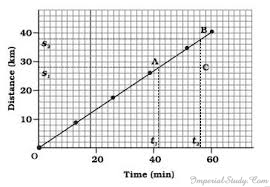Graph for non-uniform speed-

The distance-time graph for non-uniform speed is different from that of uniform motion as it is in the form of a curve. This graph shows the non-linear variation of the distance travelled by an object with time.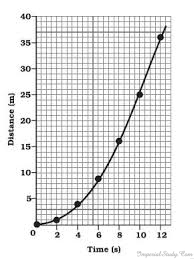8.5.2- Velocity-Time Graphs

In velocity-time graphs, the time is taken along the x-axis and the velocity is taken along the y-axis. Now, let us discuss about velocity-time graphs for uniform and non-uniform velocity:

Graph for uniform velocity-

In case of uniform velocity, the height of the velocity-time graph will not change with time. It will be a straight line parallel to the x-axis.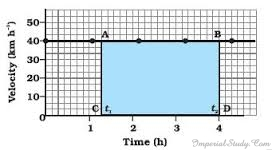The product of the velocity and time gives the displacement of an object which is moving with a uniform velocity. So, in our graph, the area enclosed by the velocity-time graph and the time axis will be equal to the magnitude of displacement. In above graph, the distance moved by the object in time (t2-t­1) is equal to AC ×CD;

= [(40 km/h) × (t2-t1) h]

= 40 (t2-t1) km

= area of rectangle ABCD which is shaded in blue colour

Graph for uniformly accelerated motion-

Before moving to non-uniform motion, it is important to discuss about the velocity-time graph for a uniformly accelerated motion of an object. In such cases, for example when a car is moving with uniformly accelerated velocity, the velocity changes by equal amounts in equal intervals of time. So, in every case of uniformly accelerated motion, the velocity-time graph is a straight line.

In the following graph, we can determine the distance travelled by the object by calculating the area of figure ABCDE under the velocity-time graph that is given below.

i.e. the distance, s = area ABCDE

= area of rectangle ABCD + area of triangle ADE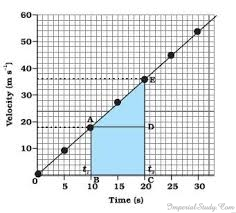= [AB × BC] + [½(AD × DE)]

Graph for non-uniform velocity-

For non-uniform velocity, there is not much thing to look at except to see how these graphs do look. There are two types of such graphs available:

1. Motion of an object whose velocity is decreasing with time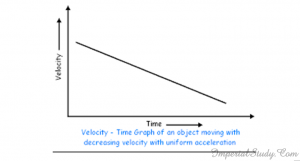As you can see, there is not much thing to discuss about this graph as this graph is self-explanatory.

2. Non-uniform variation of velocity of an object with time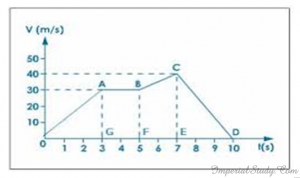However, in the above graph you can see that the non-uniform motion has been represented by zigzag lines which is divided into several parts. You can easily find out the magnitude of distance by calculating Area of ( triangle AOG + rectangle ABFG + trapezium BCEF + triangle CDE ).

8.6 – Equations of Motion using the Graphical Method

When an object is moving along a straight line with uniform acceleration, then its velocity, acceleration during the motion and the distance covered by it in a certain time interval can be related with the help of certain equations which are known as the equations of motion. These equations are:

v = u + at         —- (i)

s = ut + ½ at2           —- (ii)

2 a s = v2 – u2                —- (iii)

where,

u – initial velocity                                     v – final velocity

a – uniform acceleration                           s – distance travelled

t – time taken

These three equations can be represented using the graph which is given below: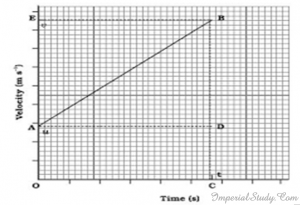8.6.1- Equation for Velocity-Time Relation

In the graph given above, consider a body moving with a uniform acceleration a, with the initial velocity u and the final velocity v in the time t. The perpendicular lines BC and BE are drawn from B to x-axis and y-axis. The initial velocity is OA and final velocity is BC. Also, the time taken is OC. AD || OC.

Now,

BC = BD + DC

= BD + OA

Substituting BC = v and OA = u,

v = BD + u

Or,                                        BD = vu

Acceleration =                 Change in velocity/Time

Or, a = (v – u)/t

v – u = at

Or, v = u + at

8.6.2- Equation for Position-Time Relation

Now, again in the same graph we can take the distance s as:

s = area of OABC (which is a trapezium)

= OA × OC + ½ (AD × BD)

Now, substituting OA = u, OC = AO = t

BD = v – u = at

s = u × t + 1/2 (AD × BD)

s = ut + ½ (t × at)

or, s = ut + ½ at2

8.6.3- Equation for Position-Velocity Relation

Again in the same graph, we have,

s = area of trapezium OABC

= (OA +BC) × OC/2

Substituting OA = u, BC = v and OC = t,

s = (u+v) t/2

From velocity-time relation,

t = (v-u)/a

s = (v+u) × (v-u)/2a

Or,      2as = v2– u2

8.7- Uniform Circular Motion

Now we have come to the last topic of our first physics chapter of class 9th i.e. uniform circular motion. Since there are a lot of questions asked about this topic in exams, we must discuss this whole chapter little by little breaking it into various subtopics. Let us first start with the definition of this term itself:

Uniform Circular Motion:

The motion in a circular path with uniform speed is known as uniform circular motion. Thus, an object moving in a circular path with uniform speed is said to be moving in uniform circular motion.

Now let us move to further discussions:

Changing of directions during circular motion:

Suppose an object moves on a rectangular path, then the object has to change its direction four times before it completes its path. Similarly, in a hexagon, it will change its direction six times and in a octagon, it will change its direction eight times. If we go on increasing the number of sides indefinitely, the shape of the path would slowly approach the shape of a circle and the length of the sides would be decreased into a point. Also, an object that is moving along a circular path would change its direction an indefinite number of times as the number of sides is increased by an indefinite number.

Change of velocity during circular motion:

When an object moves along a circular path, the only change in its velocity is due to the change in the direction of motion. Thus, the motion of an object moving along a circular path is also an example of accelerated motion.

Calculating the velocity during motion on a circular path:

Suppose the radius of the circular path is r, then its circumference is given by 2πr. If an object takes t seconds to go once around the circular path, the velocity v is given by

v =           2πr/2

Example of uniform circular motion:

If you take a piece of thread and tie a small piece of stone at one of its ends, then hold the other end of the thread in one hand and move the thread in a circular motion, it will be an example of an object moving in uniform circular motion. If, at any instance, you release the thread from your hand, the thread would move in a straight line which shows that the direction of motion changed at every point when the stone was moving along the circular path.

Other examples of uniform circular motion are the movement of moon and the earth, a satellite in a circular orbit around the earth, a cyclist on a circular track at constant speed and an athlete rotating his body before throwing a hammer or discuss.

So, here our first physics chapter of Motion for class 9th comes to an end. We have discussed all important topics and you need to be clear in all these topics because these are simply the basic topics and would be useful in higher studies as the higher studies are all based on these small topics that you learn in the small classes. If you have any other doubt, leave a comment or contact us using our e-mails provided in our site or go to hello@imperialstudy.com .